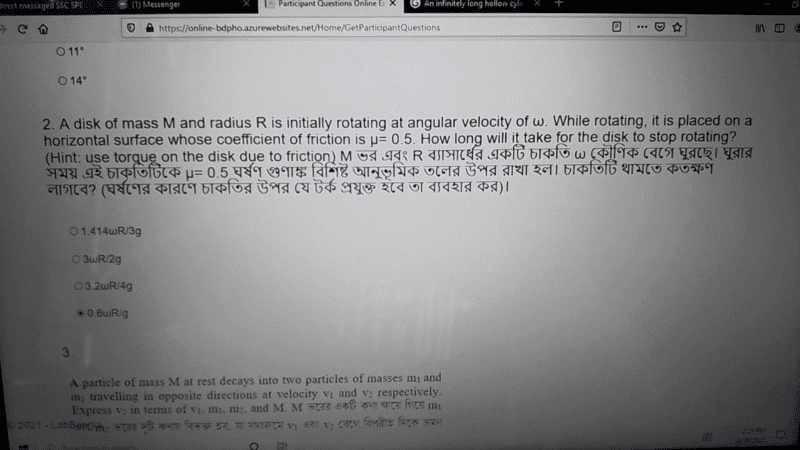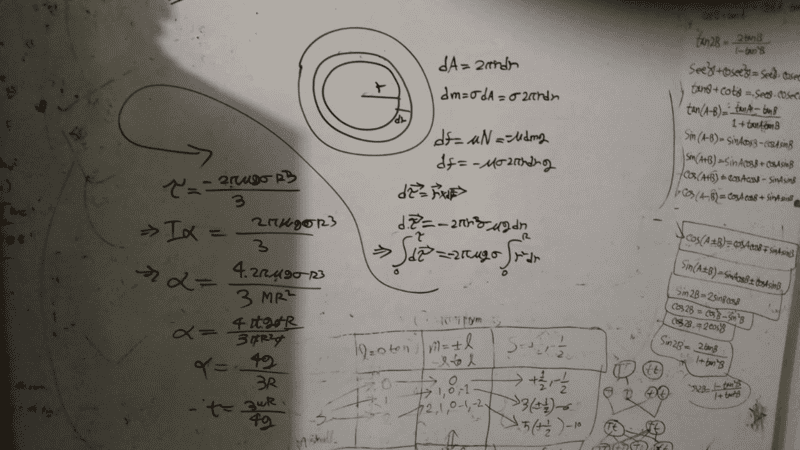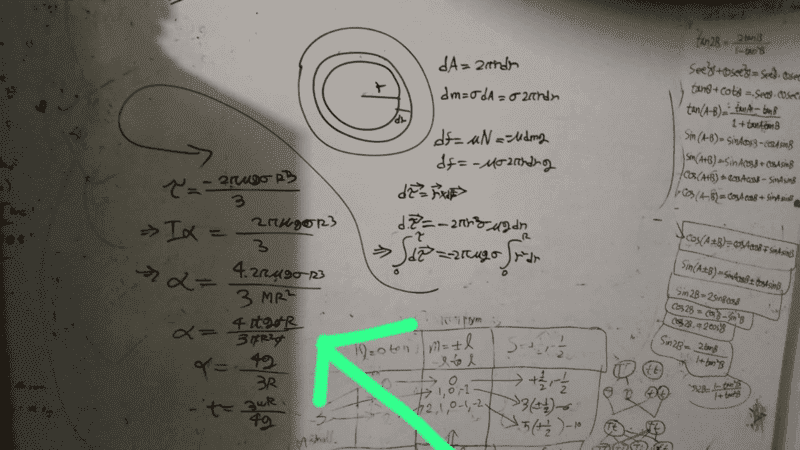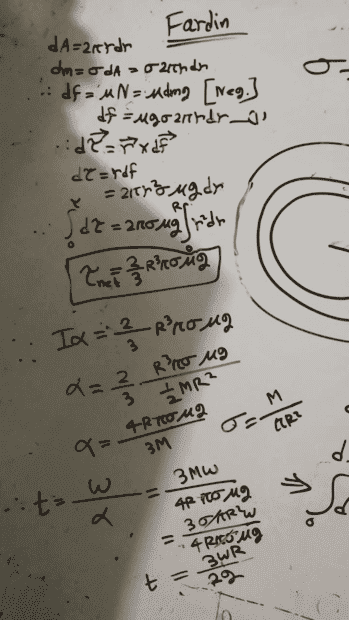# How long does it take for the disk to stop rotating?

Istiak
Homework Statement:
A disk of masses M and radius R is initially rotating at angular velocity \omega. While rotating, it is placed on a horizontal surface whose coefficient of friction is \mu =0.5 How long take for the disk to stop rotating?
Relevant Equations:
\tau = r . F
Question :Solution attempt :for

Homework Helper
Gold Member
2022 Award
Are you asking us to check your working because it does not give one of the answers in the list?

Your writing/working is difficult to read and follow. But as far as I can tell you have made a simple error: you appear to have substituted μ=1 rather than μ=0.5

•Delta2
Homework Helper
Gold Member
You have made a simple algebraic error. The moment of inertial of a solid disk with respect to the z-axis is ##\frac{1}{2}MR^2##. So when you divide by this to find the angular acceleration you should get $$a=\frac{4\pi\mu g\sigma R^3}{3MR^2}$$, but you have keep an additional factor of 2 (which later you get rid by replacing ##\mu=0.5##). So after simplifications you actually get $$a=\frac{2g}{3R}$$ which lead you to one of the available options.

•Steve4Physics
Istiak
Are you asking us to check your working because it does not give one of the answers in the list?

Your writing/working is difficult to read and follow. But as far as I can tell you have made a simple error: you appear to have substituted μ=1 rather than μ=0.5
2×0.5=1

Istiak
You have made a simple algebraic error. The moment of inertial of a solid disk with respect to the z-axis is ##\frac{1}{2}MR^2##. So when you divide by this to find the angular acceleration you should get $$a=\frac{4\pi\mu g\sigma R^3}{3MR^2}$$, but you have keep an additional factor of 2 (which later you get rid by replacing ##\mu=0.5##). So after simplifications you actually get $$a=\frac{2g}{3R}$$ which lead you to one of the available options.Homework Helper
Gold Member
Your error is one line above from where your green arrow is pointing. You write that $$a=\frac{4 \cdot 2 \pi\mu g\sigma R^3}{3MR^2}$$ while the correct is (as i said at my earlier post) that $$a=\frac{4\pi\mu g\sigma R^3}{3MR^2}$$

•Steve4Physics
Homework Helper
Gold Member
Let me ask you one straight question, what do you take it to be the moment of inertia of the solid disk? $$\frac{1}{2}MR^2$$ OR $$\frac{1}{4}MR^2$$?
MoI around the z axis (perpendicular to the plane of the disk) is the first, while MoI around x or y-axis is the second...

Istiak
Your error is one line above from where your green arrow is pointing. You write that $$a=\frac{4 \cdot 2 \pi\mu g\sigma R^3}{3MR^2}$$ while the correct is (as i said at my earlier post) that $$a=\frac{4\pi\mu g\sigma R^3}{3MR^2}$$
Why there won't be 4 times 2?

Homework Helper
Gold Member
Why there won't be 4 times 2?
Because the Moment of Inertia of the solid disk (around the z-axis perpendicular to xy plane-the plane of the disk) is ##I=\frac{1}{2}MR^2## so you actually have ##2\cdot 2=4## there and NOT ##4\cdot 2=8##...

Homework Helper
Gold Member
2022 Award
2×0.5=1
So I believe.

You have made an error involving a factor of 2. It might be (as @Delta2 notes) something to do with the ½ in ½MR², or as I note, something to do with the value of μ (=0.5). Maybe it's a mixture of the two - e.g. incorrectly mixing the two ½s.

For me, the main problem is that your hand-writing is very difficult to read, so it's hard to be certain exactly what you've done.

But the only 2 things you now need to do are:
1) carefully repeat your working (say from 'Iα =' onwards) to locate your mistake;
2) click 'Likes' for @Delta2 and me to acknowledge the time/effort/help we have supplied!

•Delta2
Istiak
So I believe.

You have made an error involving a factor of 2. It might be (as @Delta2 notes) something to do with the ½ in ½MR², or as I note, something to do with the value of μ (=0.5). Maybe it's a mixture of the two - e.g. incorrectly mixing the two ½s.

For me, the main problem is that your hand-writing is very difficult to read, so it's hard to be certain exactly what you've done.

But the only 2 things you now need to do are:
1) carefully repeat your working (say from 'Iα =' onwards) to locate your mistake;
2) click 'Likes' for @Delta2 and me to acknowledge the time/effort/help we have supplied!Is it OK now?

•Delta2
Homework Helper
Gold Member
YES!

Homework Helper
Gold Member
2022 Award
View attachment 285452

Is it OK now?
That's the same answer as I get - so I'd say yes! The important thing is that you found/understood where you made the mistake.

•Istiak